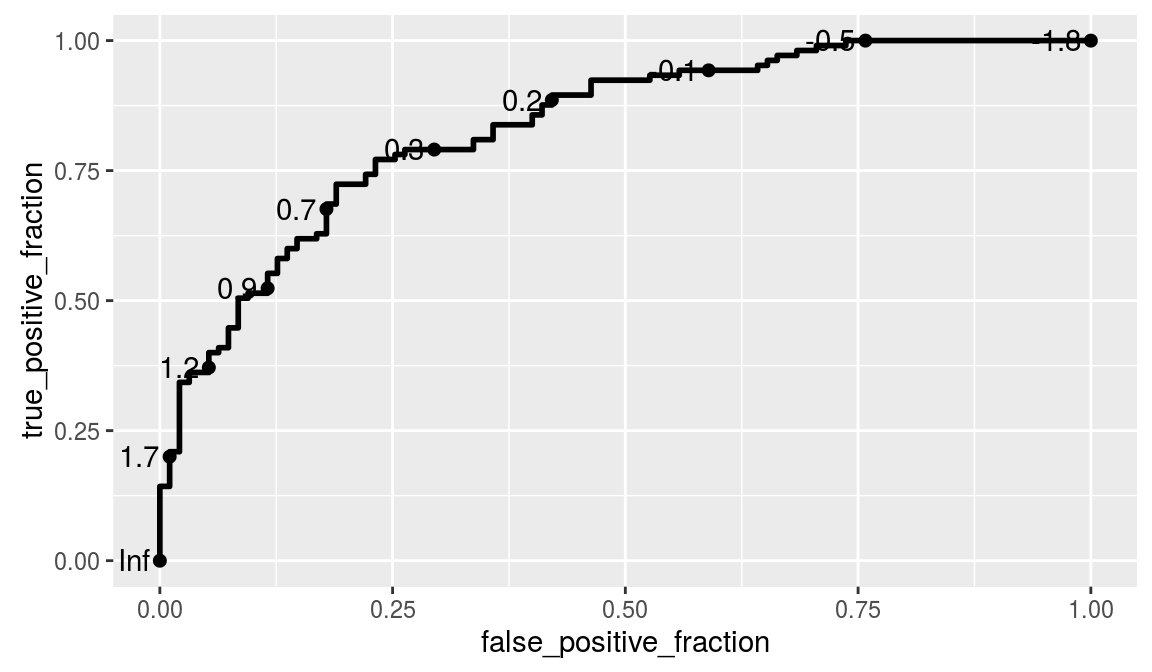ggplot2 now has an official extension mechanism. This means that others can now easily create their own stats, geoms and positions, and provide them in other packages. This should allow the ggplot2 community to flourish, even as less development work happens in ggplot2 itself. This page showcases these extensions.

### plotROC

https://github.com/sachsmc/plotROC

Most ROC curve plots obscure the cutoff values and inhibit interpretation and comparison of multiple curves. This attempts to address those shortcomings by providing plotting and interactive tools. Functions are provided to generate an interactive ROC curve plot for web use, and print versions

``````# Example from http://sachsmc.github.io/plotROC/
library(ggplot2)
library(plotROC)

# I start by creating an example data set. There are 2 markers,
# one that is moderately predictive and one that is not as predictive.

set.seed(2529)
D.ex <- rbinom(200, size = 1, prob = .5)
M1 <- rnorm(200, mean = D.ex, sd = .65)
M2 <- rnorm(200, mean = D.ex, sd = 1.5)

test <- data.frame(D = D.ex, D.str = c("Healthy", "Ill")[D.ex + 1],
M1 = M1, M2 = M2, stringsAsFactors = FALSE)

# The Roc Geom
# Next I use the ggplot function to define the aesthetics, and
# the geom_roc function to add an ROC curve layer.

basicplot <- ggplot(test, aes(d = D, m = M1)) + geom_roc()
basicplot``````